2022年5月27日 星期五

# 《像素神庙》极限立体布局分享

### 《英雄联盟手游》德玛西亚皇子嘉文四世天赋加点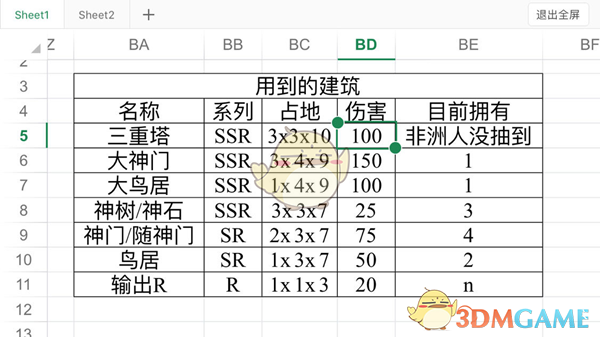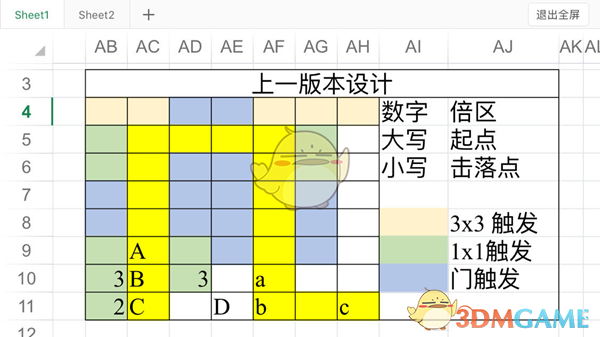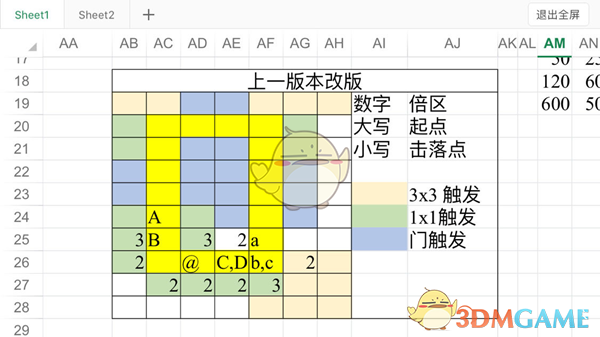(门)(100×5+50×2)x3 = 1800

(3×3)100x2x3 = 600，2倍区换1×1 = 20

(R)20x(6×3+3×2+4×1)= 560

(门)(100×1+150×1+50×2+20×2)x3 = 1170

(3×3)175(R)560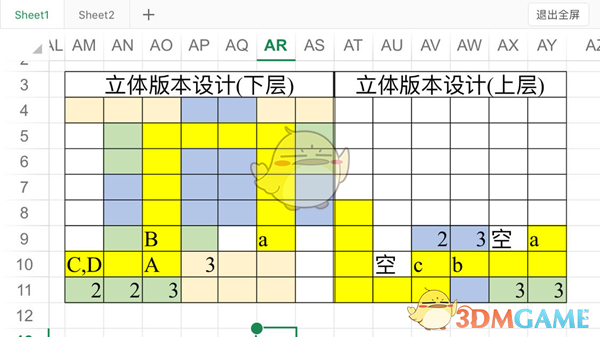(门)(100×4+50×2)x3+150x1x2 = 1800

(3×3)100x2x3 = 600，3倍区换1×1 = 20×2 = 40

(R)20x(6×3+3×2+2×1)= 520

*CD另一侧因为和4倍区自然植物位置冲突无法放置，如不放植物则总伤害相对较低

(门)(100×1+50×2+20×6)x3 + 150×2 = 1260

(3×3)200 (R)500

(考虑到宝玉数量限制，在不氪金的前提下，2×3突破后的神门能大于4个1×1 R实际伤害)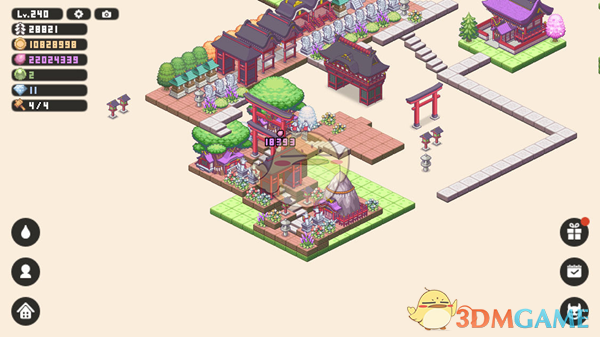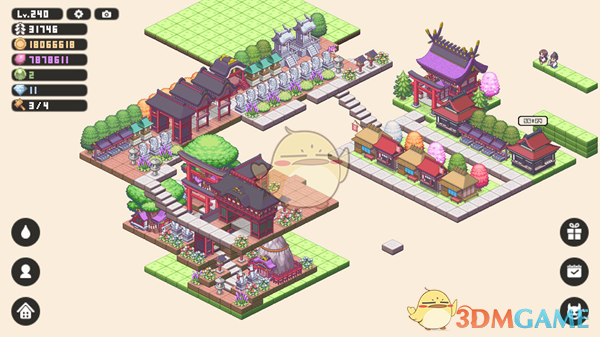5个SR神门，两个SSR神门20，主要的几个R15，其它都是10，就可以上275。

### 《三国志战略版》S12铁骑吴骑开荒攻略

【铁骑吴骑】 由于吴骑的优越性，很...